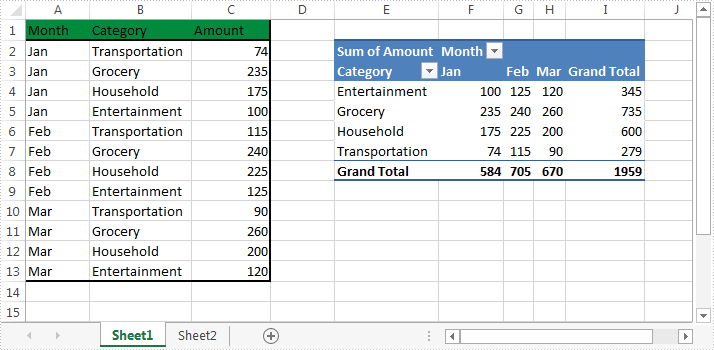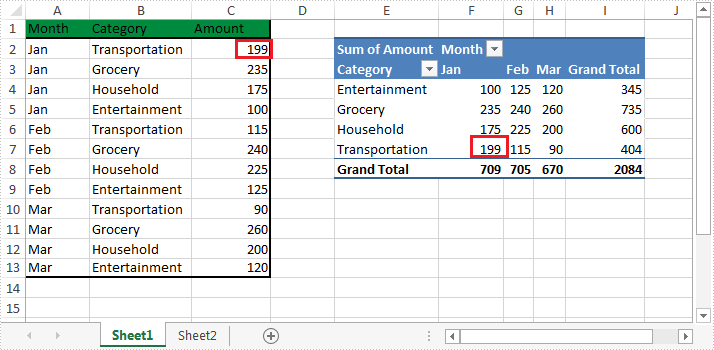# Refresh PivotTable in Excel in C#

By default, the data source of PivotTable won't be refreshed automatically. If we update the data source of our PivotTable, the PivotTable that was built on that data source needs to be refreshed. This article is going to elaborate how to refresh PivotTable in Excel programmatically in c# using Spire.XLS.

Below is the screenshot of the example Excel file:Detail steps:

Step 1: Instantiate a Workbook object and load the Excel file.

```Workbook workbook = new Workbook();
```

Step 2: Get the first worksheet.

```Worksheet sheet = workbook.Worksheets;
```

Step 3: update the data source of PivotTable.

```sheet.Range["C2"].Value = "199";
```

Step 4: Get the PivotTable that was built on the data source.

```XlsPivotTable pt = workbook.Worksheets.PivotTables as XlsPivotTable;
```

Step 5: Refresh the data of PivotTable.

```pt.Cache.IsRefreshOnLoad = true;
```

Step 6: Save the file.

```workbook.SaveToFile("Output.xlsx", ExcelVersion.Version2013);
```

Output after updating data source and refreshing the PivotTable:Full code:

```using Spire.Xls;

namespace Refresh_Pivot_Table_in_Excel
{
class Program
{
static void Main(string[] args)
{
//Instantiate a Workbook object
Workbook workbook = new Workbook();

//Get the first worksheet
Worksheet sheet = workbook.Worksheets;

//Update the data source of PivotTable
sheet.Range["C2"].Value = "199";

//Get the PivotTable that was built on the data source
XlsPivotTable pt = workbook.Worksheets.PivotTables as XlsPivotTable;

//Refresh the data of PivotTable